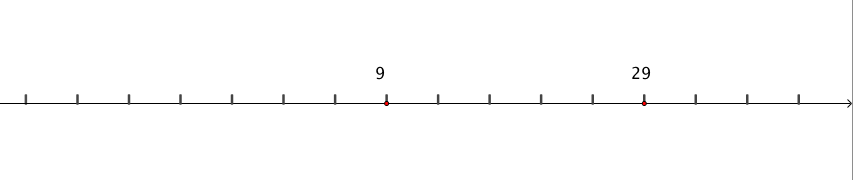#### You may also like### Worms

Place this "worm" on the 100 square and find the total of the four squares it covers. Keeping its head in the same place, what other totals can you make?### Greetings

From a group of any 4 students in a class of 30, each has exchanged Christmas cards with the other three. Show that some students have exchanged cards with all the other students in the class. How many such students are there?### Writ Large

Suppose you had to begin the never ending task of writing out the natural numbers: 1, 2, 3, 4, 5.... and so on. What would be the 1000th digit you would write down.

# Number Lines in Disguise

### Why do this problem?

Looking at a familiar context in a much more challenging scenario can pique curiosity. Number lines are used a lot when children are developing number sense and then are revisited when drawing axes for graphs. This is an opportunity to explore number lines in a challenging context.

This problem also gives us an opportunity to show some strategies we might encourage when students are stuck. Students can pick the level they wish to work at and we can use this to demonstrate when it is useful to try simpler examples to help us develop an approach to solving a more difficult problem.

### Possible approach

Begin by showing students this image. (PowerPoint Slide)"Have a look at this number line. What do you notice?"
Give students a short time to look at the image in silence, and then invite them to discuss with their neighbour.

"Can you determine the value of other points on the line? How do you know?"

If computers are available, this would be a good time to introduce the interactivity.
"Work with a partner to explore some other examples, and see if you can find a strategy that will work for any level." Alternatively, you may find this worksheet useful, which contains a few examples from each level of the interactivity. (Thank you to Mr Gray who created the sheet and very kindly shared it with us. He reported that he found it particularly useful as students were able to record their thinking on it.)

Level 1 = whole number steps, small negative numbers possible
Level 2 = fractional steps (halves quarters and eigths only), small negative numbers possible
Level 3 = fractional steps (any), small negative numbers
Level 4 = fractional steps (any), negative numbers are always included in the number line
Level 5 = like Level 4 but with larger numbers

While students are working, circulate and listen for pairs who are developing strategies or who have particularly useful insights. Once most students have successfully solved a number of examples, bring the class together and invite pairs to share their strategies and insights.

### Key questions

Can you find the value of any of the points on the line?
Where is $0$ on the line?
How do you know?
Did you always use the same strategy?
Can you find a strategy that you can use for any example?